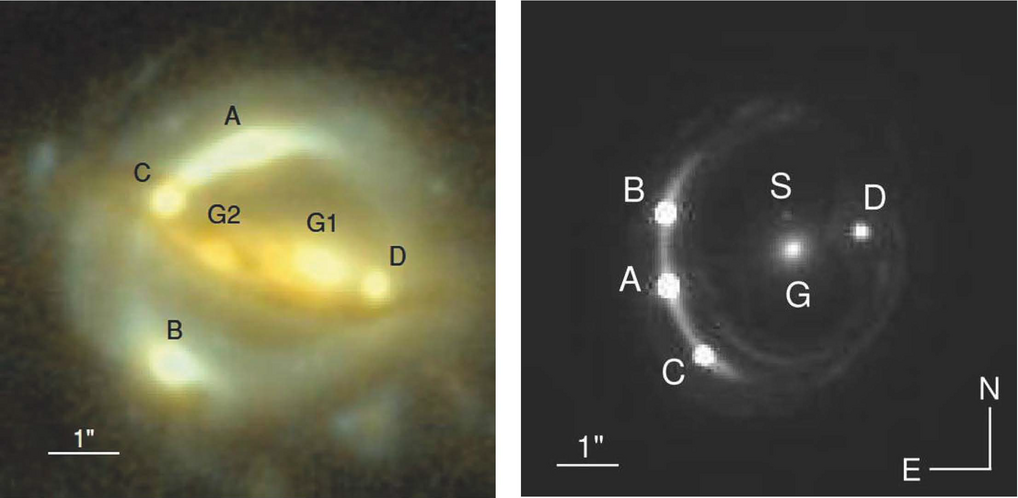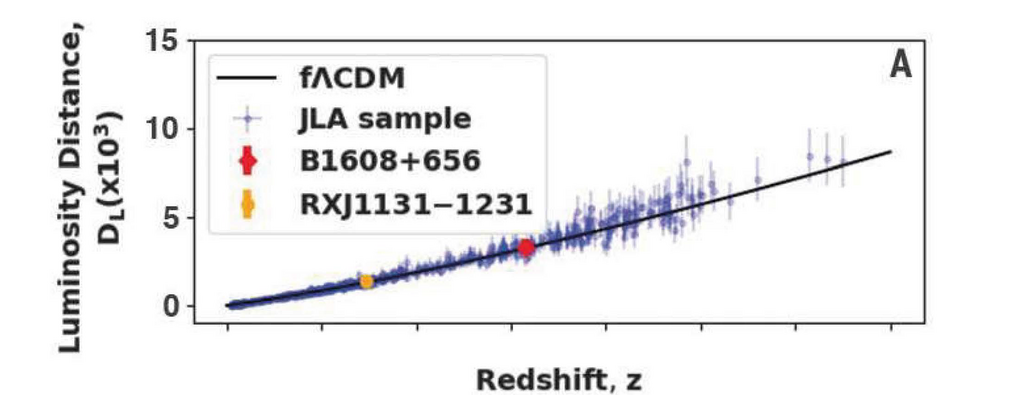# High value for Hubble constant

Astronomers measure the expansion of the universe with the help of gravitational lenses

September 13, 2019

The expansion rate of the Universe today is described by the so-called Hubble constant and different techniques have come to inconsistent results about how fast our Universe actually does expand. An international team led by the Max Planck Institute for Astrophysics (MPA) has now used two gravitational lenses as new tools to calibrate the distances to hundreds of observed supernovae and thus measure a fairly high value for the Hubble constant. While the uncertainty is still relatively large, this is higher than that inferred from the cosmic microwave background.Images of the two lensing systems used in this study, B1608+656 and RXJ1131. Labels A to D denote images of the background quasar, G1 and G2 are lens galaxies on the left, G is the lens galaxy on the right with a satellite galaxy S. © MPA

Gravitational lensing describes the fact that light is deflected by large masses in the Universe, just like a glass lens will bend a light right on Earth. In recent years, cosmologists have increasingly used this effect to measure distances by exploiting the fact that, in a multiple image system, an observer will see photons arriving from different directions at different times due to the difference in optical path lengths for the various images. This measurement thus gives a physical size of the lens, and comparing it to an observed size in the sky gives a geometric distance estimate called the “angular diameter distance”. Such distance measurements in astronomy are the basis for measurements of the Hubble constant, named after the astronomer Edwin Hubble, who found a linear relationship between the redshifts (and thus the expansion velocity of the Universe) and the distances of galaxies (which was also independently found by Georges Lemaître).

“There are multiple ways to measure distances in the Universe, based on our knowledge of the object whose distance is being measured,” explains Sherry Suyu (MPA/TUM), who is a world expert in using gravitational lensing for determining the Hubble constant. “A well-known technique is the luminosity distance using supernovae explosions; however, they must adopt an external calibrator of the absolute distance scale. With our analysis of gravitational lens systems we can provide a completely new, independent anchor for this method.”

The team used two strong gravitational lens systems B1608+656 and RXJ1131 (see Figure 1). In each of these systems, there are four images of a background galaxy with one or two foreground galaxies acting as lenses. This relatively simple configuration allowed the scientists to produce an accurate lensing model and thus measure the angular diameter distances to a precision of 12 to 20% per lens. These distances were then applied as anchors to 740 supernovae in a public catalogue (Joint Light-curve Analysis dataset).Derived Hubble diagram, using the two lens systems (red and yellow dots) as anchors for the 740 supernovae in the JLA dataset. © MPA

“By construction, our method is insensitive to the details of the assumed cosmological model,” states Inh Jee (MPA), who did the statistical analysis and combined the supernova data with the lensing distances. “We get a fairly high result for the Hubble constant and although our measurement has a larger uncertainty than other direct methods, this is dominated by statistical uncertainty because we use only two lens systems.”

The value for the Hubble constant based on this new analysis is about 82 +/- 8 km/s/Mpc. This is consistent with values derived from the distance ladder method, which uses different anchors for the supernova data, as well as with values from time-delay distances, where other gravitational lensing systems were used to determine the Hubble constant directly.

“Again this new measurement confirms that there seems to be a systematic difference in values for the Hubble constant derived directly from local or intermediate sources and indirectly from the cosmic microwave background,” states Eiichiro Komatsu, director at MPA, who oversaw this project. “If confirmed by further measurements, this discrepancy would call for a revision of the standard model of cosmology.”

HAE

Go to Editor View